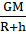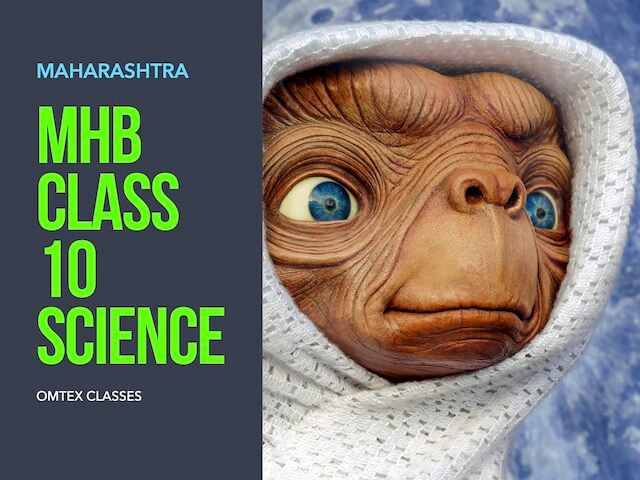### State with reasons whether the following sentences are true or false a. If a spacecraft has to be sent away from the influence of earth’s gravitational field, its velocity must be less than the escape velocity.

Question 2.

State with reasons whether the following sentences are true or false

a. If a spacecraft has to be sent away from the influence of earth’s gravitational field, its velocity must be less than the escape velocity.

b. The escape velocity on the moon is less than that on the earth.

c. A satellite needs a specific velocity to revolve in a specific orbit.

d. If the height of the orbit of a satellite increases, its velocity must also increase.

Answer:

(a) False

Explanation: Spacecrafts should escape the earth’s gravitational force to travel to outer space. Escape velocity is the minimum speed required by an object i.e. satellite to escape from the gravitational force of the earth. So its speed should be more than the escape velocity.

(b) True

Explanation: The mass of moon is less than the mass of earth so its gravitational pull is less hence escape velocity on moon is less as compared to earth.

(c) True

Explanation: A satellite requires a specific velocity to revolve in a specific orbit called critical velocity which acts as a tangential direction to the orbit.

(d) False

Explanation: If the height of the orbit of a satellite increases, its velocity decreases. As tangential velocity of satellite is inversely proportional to the height of orbit of satellite from earth’s surface.

V= √Where V = tangential velocity

G = gravitational constant

M = mass of earth

R = radius of earth

h = height of satellite above earth surface